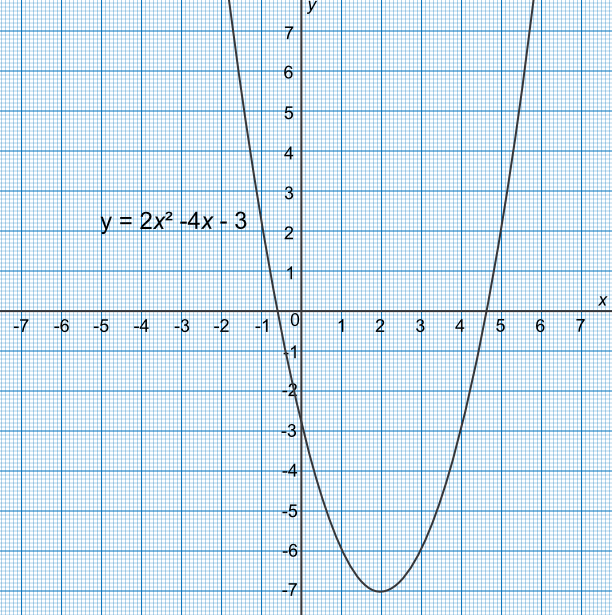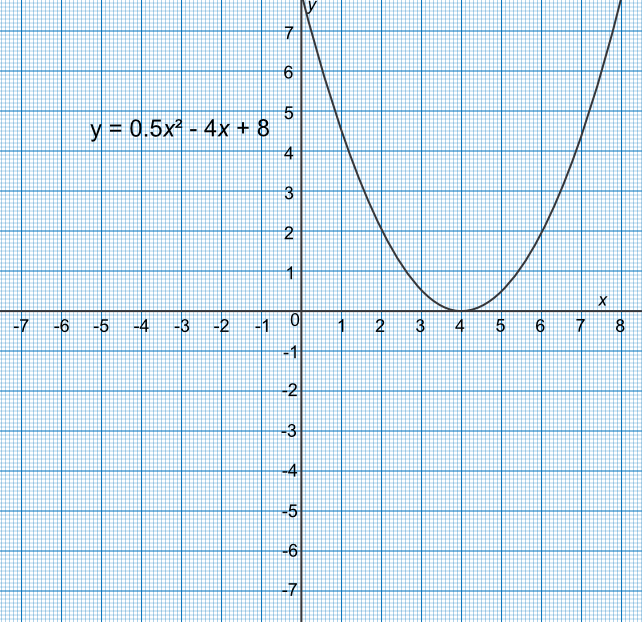Solving Quadratic Equations using a Graph

## Solving Quadratic Equations using a Graph

Graphs can be used to solve quadratic equations. Arrange the equation so that it is equal to zero on one side. Plot the graph as y against the function. The solutions can be found where the line crosses the x-axis.

Note that there may be 0, 1 or 2 solutions, depending on how many times the graph crosses the axis.

Note that the answer may only be approximate, depending on how accurately the graph is drawn.

Exam Tip: Substitute the values of x from the graph and substitute into the original equation to check the accuracy of the measurement.

## Example 1

By drawing a graph for -2 ≤ x ≤ 6, estimate the solutions to the equation x^2 - 4x - 3 = 0 to one decimal place.

Plot the graph: coordinates are (-2, 9), (-1, 2), (0, -3), (1, -6), (2, -7), (3, -6), (4, -3), (5, 2), (6, 9)

Graph crosses the x-axis at 4.6 and -1.6.Check: (4.6)^2 - 4(4.6) - 3 = -0.24(-0.6)^2 - 4(-0.6) - 3 = -0.24

Answer: x=4.6 text( and ) x= -0.6

## Example 2

By drawing a graph for 0 ≤ x ≤ 8, estimate the solutions to x^2-8x=-16.

Rearrange the equation to 0.5x^2-4x+8=0, and plot the graph as y=x^2-8x+16.

Points to plot are (0,8), (1,4.5), (2,2), (3,0.5), (4,0), (5,0.5), (6,2), (7,4.5), (8,8)

Graph touches the x-axis at x=4. Note that there are two equal roots.Check:

0.5(4)^2 - 4(4) + 8 = 0

Answer: x=4 (both roots take the same value)